# WaterPropsIAPWS Class Reference [Thermodynamic Properties]

Class for calculating the equation of state of water. More...

#include <WaterPropsIAPWS.h>

Collaboration diagram for WaterPropsIAPWS: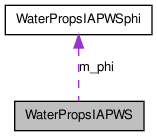[legend]

List of all members.

## Public Member Functions

WaterPropsIAPWS ()
Base constructor.
WaterPropsIAPWS (const WaterPropsIAPWS &right)
Copy constructor.
WaterPropsIAPWSoperator= (const WaterPropsIAPWS &right)
assignment constructor
~WaterPropsIAPWS ()
destructor
void setState_TR (doublereal temperature, doublereal rho)
Set the internal state of the object wrt temperature and density.
doublereal helmholtzFE () const
Calculate the Helmholtz free energy in mks units of J kmol-1 K-1, using the last temperature and density.
doublereal Gibbs () const
Calculate the Gibbs free energy in mks units of J kmol-1 K-1.
doublereal enthalpy () const
Calculate the enthalpy in mks units of J kmol-1 using the last temperature and density.
doublereal intEnergy () const
Calculate the internal energy in mks units of J kmol-1.
doublereal entropy () const
Calculate the entropy in mks units of J kmol-1 K-1.
doublereal cv () const
Calculate the constant volume heat capacity in mks units of J kmol-1 K-1 at the last temperature and density.
doublereal cp () const
Calculate the constant pressure heat capacity in mks units of J kmol-1 K-1 at the last temperature and density.
doublereal molarVolume () const
Calculate the molar volume (kmol m-3) at the last temperature and density.
doublereal pressure () const
Calculates the pressure (Pascals), given the current value of the temperature and density.
doublereal density (doublereal temperature, doublereal pressure, int phase=-1, doublereal rhoguess=-1.0)
Calculates the density given the temperature and the pressure, and a guess at the density.
doublereal density_const (doublereal pressure, int phase=-1, doublereal rhoguess=-1.0) const
Calculates the density given the temperature and the pressure, and a guess at the density, while not changing the internal state.
doublereal density () const
Returns the density (kg m-3).
doublereal temperature () const
Returns the temperature (Kelvin).
doublereal coeffThermExp () const
Returns the coefficient of thermal expansion.
doublereal coeffPresExp () const
Returns the isochoric pressure derivative wrt temperature.
doublereal isothermalCompressibility () const
Returns the coefficient of isothermal compressibility for the state of the object.
doublereal dpdrho () const
Returns the value of dp / drho at constant T for the state of the object.
doublereal psat_est (doublereal temperature) const
This function returns an estimated value for the saturation pressure.
doublereal psat (doublereal temperature, int waterState=WATER_LIQUID)
This function returns the saturation pressure given the temperature as an input parameter, and sets the internal state to the saturated conditions.
doublereal densSpinodalWater () const
Return the value of the density at the water spinodal point (on the liquid side) for the current temperature.
doublereal densSpinodalSteam () const
Return the value of the density at the water spinodal point (on the gas side) for the current temperature.
int phaseState (bool checkState=false) const
Returns the Phase State flag for the current state of the object.
doublereal Tcrit () const
Returns the critical temperature of water (Kelvin).
doublereal Pcrit () const
Returns the critical pressure of water (22.064E6 Pa).
doublereal Rhocrit () const
Return the critical density of water (kg m-3).

## Private Member Functions

void calcDim (doublereal temperature, doublereal rho)
Calculate the dimensionless temp and rho and store internally.
void corr (doublereal temperature, doublereal pressure, doublereal &densLiq, doublereal &densGas, doublereal &delGRT)
Utility routine in the calculation of the saturation pressure.
void corr1 (doublereal temperature, doublereal pressure, doublereal &densLiq, doublereal &densGas, doublereal &pcorr)
Utility routine in the calculation of the saturation pressure.

## Private Attributes

WaterPropsIAPWSphim_phi
pointer to the underlying object that does the calculations.
doublereal tau
Dimensionless temperature.
doublereal delta
Dimensionless density.
int iState
Current state of the system.

## Detailed Description

Class for calculating the equation of state of water.

The reference is W. Wagner, A. Prub, "The IAPWS Formulation 1995 for the Themodynamic Properties of Ordinary Water Substance for General and Scientific Use," J. Phys. Chem. Ref. Dat, 31, 387, 2002.

This class provides a very complicated polynomial for the specific helmholtz free energy of water, as a function of temperature and density.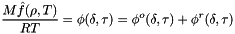where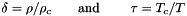The following constants are assumed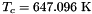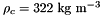The free energy is a unique single-valued function of the temperature and density over its entire range.

Note, the base thermodynamic state for this class is the one used in the steam tables, i.e., the liquid at the triple point for water has the following properties:

• u(273.16, rho) = 0.0
• s(273.16, rho) = 0.0
• psat(273.16) = 611.655 Pascal
• rho(273.16, psat) = 999.793 kg m-3

Therefore, to use this class within Cantera, offsets to u() and s() must be used to put the water class onto the same basis as other thermodynamic quantities. For example, in the WaterSSTP class, these offsets are calculated in the following way. The thermodynamic base state for water is set to the NIST basis here by specifying constants EW_Offset and SW_Offset. These offsets are calculated on the fly so that the following properties hold:

• Delta_Hfo_idealGas(298.15, 1bar) = -241.826 kJ/gmol
• So_idealGas(298.15, 1bar) = 188.835 J/gmolK

The offsets are calculated by actually computing the above quantities and then calculating the correction factor.

This class provides an interface to the WaterPropsIAPWSphi class, which actually calculates the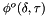and the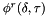polynomials in dimensionless form.

All thermodynamic results from this class are returned in dimensional form. This is because the gas constant (and molecular weight) used within this class is allowed to be potentially different than that used elsewhere in Cantera. Therefore, everything has to be in dimensional units. Note, however, the thermodynamic basis is set to that used in the steam tables. (u = s = 0 for liquid water at the triple point).

This class is not a ThermoPhase. However, it does maintain an internal state of the object that is dependent on temperature and density. The internal state is characterized by an internally storredand a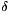value, and an iState value, which indicates whether the point is a liquid, a gas, or a supercritical fluid. Along with that theand avalues are polynomials ofand athat are kept by the WaterPropsIAPWSphi class. Therefore, wheneveroris changed, the function setState() must be called in order for the internal state to be kept up to date.

The class is pretty straightfoward. However, one function deserves mention. the density() function calculates the density that is consistent with a particular value of the temperature and pressure. It may therefore be multivalued or potentially there may be no answer from this function. It therefore takes a phase guess and a density guess as optional parameters. If no guesses are supplied to density(), a gas phase guess is assumed. This may or may not be what is wanted. Therefore, density() should usually at leat be supplied with a phase guess so that it may manufacture an appropriate density guess. density() manufactures the initial density guess, nondimensionalizes everything, and then calls WaterPropsIAPWSphi::dfind(), which does the iterative calculation to find the density condition that matches the desired input pressure.

The phase guess defines are located in the .h file. they are

• WATER_GAS
• WATER_LIQUID
• WATER_SUPERCRIT

There are only three functions which actually change the value of the internal state of this object after it's been instantiated

• setState_TR(temperature, rho)
• density(temperature, pressure, phase, rhoguess)
• psat(temperature, waterState);

The setState_TR() is the main function that sets the temperature and rho value. The density() function serves as a setState_TP() function, in that it sets internal state to a temperature and pressure. However, note that this is potentially multivalued. Therefore, we need to supply in addition a phase guess and a rho guess to the input temperature and pressure. The psat() function sets the internal state to the saturated liquid or saturated gas state, dependeing on the waterState parameter.

Because the underlying object WaterPropsIAPWSphi is privately held, you can be sure that the underlying state of this object doesn't change except due to the three function calls listed above.

Definition at line 161 of file WaterPropsIAPWS.h.

## Constructor & Destructor Documentation

 WaterPropsIAPWS ( )

Base constructor.

Definition at line 55 of file WaterPropsIAPWS.cpp.

References WaterPropsIAPWS::m_phi.

 WaterPropsIAPWS ( const WaterPropsIAPWS & right )

Copy constructor.

Parameters:
 right Object to be copied

Definition at line 68 of file WaterPropsIAPWS.cpp.

 ~WaterPropsIAPWS ( )

destructor

Definition at line 92 of file WaterPropsIAPWS.cpp.

References WaterPropsIAPWS::m_phi.

## Member Function Documentation

 void calcDim ( doublereal temperature, doublereal rho )  [private]

Calculate the dimensionless temp and rho and store internally.

Private routine

Parameters:
 temperature input temperature (kelvin) rho density in kg m-3

Definition at line 105 of file WaterPropsIAPWS.cpp.

Referenced by WaterPropsIAPWS::setState_TR().

 doublereal coeffPresExp ( ) const

Returns the isochoric pressure derivative wrt temperature.

beta = M / (rho * Rgas) (d (pressure) / dT) at constant rho

Note for ideal gases this is equal to one.

beta = delta (phi0_d() + phiR_d())

• tau delta (phi0_dt() + phiR_dt())

Definition at line 403 of file WaterPropsIAPWS.cpp.

Referenced by WaterPropsIAPWS::coeffThermExp(), and WaterProps::thermalConductivityWater().

 doublereal coeffThermExp ( ) const

Returns the coefficient of thermal expansion.

alpha = d (ln V) / dT at constant P.

Returns:
Returns the coefficient of thermal expansion

Definition at line 414 of file WaterPropsIAPWS.cpp.

 void corr ( doublereal temperature, doublereal pressure, doublereal & densLiq, doublereal & densGas, doublereal & delGRT )  [private]

Utility routine in the calculation of the saturation pressure.

Private routine

Parameters:
 temperature temperature (kelvin) pressure pressure (Pascal) densLiq Output density of liquid densGas output Density of gas delGRT output delGRT

Definition at line 444 of file WaterPropsIAPWS.cpp.

Referenced by WaterPropsIAPWS::psat().

 void corr1 ( doublereal temperature, doublereal pressure, doublereal & densLiq, doublereal & densGas, doublereal & pcorr )  [private]

Utility routine in the calculation of the saturation pressure.

Private routine

Parameters:
 temperature temperature (kelvin) pressure pressure (Pascal) densLiq Output density of liquid densGas output Density of gas pcorr output corrected pressure

Definition at line 479 of file WaterPropsIAPWS.cpp.

Referenced by WaterPropsIAPWS::psat().

 doublereal cp ( ) const

Calculate the constant pressure heat capacity in mks units of J kmol-1 K-1 at the last temperature and density.

Definition at line 866 of file WaterPropsIAPWS.cpp.

References WaterPropsIAPWSphi::cp_R(), and WaterPropsIAPWS::m_phi.

 doublereal cv ( ) const

Calculate the constant volume heat capacity in mks units of J kmol-1 K-1 at the last temperature and density.

Definition at line 859 of file WaterPropsIAPWS.cpp.

References WaterPropsIAPWSphi::cv_R(), and WaterPropsIAPWS::m_phi.

 doublereal density ( ) const

Returns the density (kg m-3).

The density is an independent variable in the underlying equation of state

Returns:
Returns the density (kg m-3)

Definition at line 311 of file WaterPropsIAPWS.cpp.

References WaterPropsIAPWS::delta, and Cantera::Rho_c.

Referenced by WaterPropsIAPWS::corr(), WaterPropsIAPWS::corr1(), and WaterPropsIAPWS::psat().

 doublereal density ( doublereal temperature, doublereal pressure, int phase = -1, doublereal rhoguess = -1.0 )

Calculates the density given the temperature and the pressure, and a guess at the density.

Sets the internal state.

Note, below T_c, this is a multivalued function.

The density() function calculates the density that is consistent with a particular value of the temperature and pressure. It may therefore be multivalued or potentially there may be no answer from this function. It therefore takes a phase guess and a density guess as optional parameters. If no guesses are supplied to density(), a gas phase guess is assumed. This may or may not be what is wanted. Therefore, density() should usually at leat be supplied with a phase guess so that it may manufacture an appropriate density guess. density() manufactures the initial density guess, nondimensionalizes everything, and then calls WaterPropsIAPWSphi::dfind(), which does the iterative calculation to find the density condition that matches the desired input pressure.

Parameters:
 temperature,: Kelvin pressure : Pressure in Pascals (Newton/m**2) phase : guessed phase of water : -1: no guessed phase rhoguess : guessed density of the water : -1.0 no guessed density
Returns:
Returns the density. If an error is encountered in the calculation the value of -1.0 is returned.

Definition at line 159 of file WaterPropsIAPWS.cpp.

 doublereal density_const ( doublereal pressure, int phase = -1, doublereal rhoguess = -1.0 ) const

Calculates the density given the temperature and the pressure, and a guess at the density, while not changing the internal state.

Note, below T_c, this is a multivalued function.

The density() function calculates the density that is consistent with a particular value of the temperature and pressure. It may therefore be multivalued or potentially there may be no answer from this function. It therefore takes a phase guess and a density guess as optional parameters. If no guesses are

supplied to density(), a gas phase guess is assumed. This may or may not be what is wanted. Therefore, density() should usually at leat be supplied with a phase guess so that it may manufacture an appropriate density guess. density() manufactures the initial density guess, nondimensionalizes everything, and then calls WaterPropsIAPWSphi::dfind(), which does the iterative calculation to find the density condition that matches the desired input pressure.

Parameters:
 pressure : Pressure in Pascals (Newton/m**2) phase : guessed phase of water : -1: no guessed phase rhoguess : guessed density of the water : -1.0 no guessed density
Returns:
Returns the density. If an error is encountered in the calculation the value of -1.0 is returned.

Definition at line 244 of file WaterPropsIAPWS.cpp.

Referenced by WaterPropsIAPWS::densSpinodalSteam(), and WaterPropsIAPWS::densSpinodalWater().

 doublereal densSpinodalSteam ( ) const

Return the value of the density at the water spinodal point (on the gas side) for the current temperature.

Returns:
returns the density with units of kg m-3

Definition at line 724 of file WaterPropsIAPWS.cpp.

 doublereal densSpinodalWater ( ) const

Return the value of the density at the water spinodal point (on the liquid side) for the current temperature.

Returns:
returns the density with units of kg m-3

Definition at line 628 of file WaterPropsIAPWS.cpp.

 doublereal dpdrho ( ) const

Returns the value of dp / drho at constant T for the state of the object.

units - Joules / kg

Returns:
returns dpdrho

Definition at line 387 of file WaterPropsIAPWS.cpp.

 doublereal enthalpy ( ) const

Calculate the enthalpy in mks units of J kmol-1 using the last temperature and density.

Definition at line 830 of file WaterPropsIAPWS.cpp.

 doublereal entropy ( ) const

Calculate the entropy in mks units of J kmol-1 K-1.

Definition at line 850 of file WaterPropsIAPWS.cpp.

References WaterPropsIAPWSphi::entropy_R(), and WaterPropsIAPWS::m_phi.

 doublereal Gibbs ( ) const

Calculate the Gibbs free energy in mks units of J kmol-1 K-1.

using the last temperature and density

Definition at line 423 of file WaterPropsIAPWS.cpp.

 doublereal helmholtzFE ( ) const

Calculate the Helmholtz free energy in mks units of J kmol-1 K-1, using the last temperature and density.

Definition at line 124 of file WaterPropsIAPWS.cpp.

 doublereal intEnergy ( ) const

Calculate the internal energy in mks units of J kmol-1.

Definition at line 840 of file WaterPropsIAPWS.cpp.

 doublereal isothermalCompressibility ( ) const

Returns the coefficient of isothermal compressibility for the state of the object.

kappa = - d (ln V) / dP at constant T.

units - 1/Pascal

Returns:
returns the isothermal compressibility

Definition at line 374 of file WaterPropsIAPWS.cpp.

References WaterPropsIAPWS::delta, WaterPropsIAPWS::dpdrho(), and Cantera::Rho_c.

 doublereal molarVolume ( ) const

Calculate the molar volume (kmol m-3) at the last temperature and density.

Definition at line 873 of file WaterPropsIAPWS.cpp.

References WaterPropsIAPWS::delta, and Cantera::Rho_c.

Referenced by PDSS_Water::gibbs_RT_ref().

 WaterPropsIAPWS & operator= ( const WaterPropsIAPWS & right )

assignment constructor

Parameters:
 right Object to be copied

Definition at line 82 of file WaterPropsIAPWS.cpp.

 doublereal Pcrit ( ) const [inline]

Returns the critical pressure of water (22.064E6 Pa).

This is hard coded to the value of 22.064E6 pascals

Definition at line 411 of file WaterPropsIAPWS.h.

 int phaseState ( bool checkState = false ) const

Returns the Phase State flag for the current state of the object.

Parameters:
 checkState If true, this function does a complete check to see where in paramters space we are

There are three values: WATER_GAS below the critical temperature but below the critical density WATER_LIQUID below the critical temperature but above the critical density WATER_SUPERCRIT above the critical temperature

Definition at line 579 of file WaterPropsIAPWS.cpp.

 doublereal pressure ( ) const

Calculates the pressure (Pascals), given the current value of the temperature and density.

The density is an independent variable in the underlying equation of state

Returns:
returns the pressure (Pascal)

Definition at line 137 of file WaterPropsIAPWS.cpp.

 doublereal psat ( doublereal temperature, int waterState = WATER_LIQUID )

This function returns the saturation pressure given the temperature as an input parameter, and sets the internal state to the saturated conditions.

Note this function will return the saturation pressure, given the temperature. It will then set the state of the system to the saturation condition. The input parameter waterState is used to either specify the liquid state or the gas state at the desired temperatue and saturated pressure.

If the input temperature, T, is above T_c, this routine will set the internal state to T and the pressure to P_c. Then, return P_c.

Parameters:
 temperature input temperature (kelvin) waterState integer specifying the water state
Returns:
Returns the saturation pressure units = Pascal

Definition at line 525 of file WaterPropsIAPWS.cpp.

 doublereal psat_est ( doublereal temperature ) const

This function returns an estimated value for the saturation pressure.

It does this via a polynomial fit of the vapor pressure curve. units = (Pascals)

Parameters:
 temperature Input temperature (Kelvin)
Returns:
Returns the estimated saturation pressure

Definition at line 334 of file WaterPropsIAPWS.cpp.

 doublereal Rhocrit ( ) const [inline]

Return the critical density of water (kg m-3).

This is equal to 322 kg m-3.

Definition at line 417 of file WaterPropsIAPWS.h.

 void setState_TR ( doublereal temperature, doublereal rho )

Set the internal state of the object wrt temperature and density.

Parameters:
 temperature temperature (kelvin) rho density (kg m-3)

Definition at line 821 of file WaterPropsIAPWS.cpp.

 doublereal Tcrit ( ) const [inline]

Returns the critical temperature of water (Kelvin).

This is hard coded to the value 647.096 Kelvin

Definition at line 405 of file WaterPropsIAPWS.h.

 doublereal temperature ( ) const

Returns the temperature (Kelvin).

Returns:
Returns the internally storred temperature

Definition at line 319 of file WaterPropsIAPWS.cpp.

References Cantera::T_c, and WaterPropsIAPWS::tau.

## Member Data Documentation

 doublereal delta [mutable, private]
 int iState [mutable, private]

Current state of the system.

Definition at line 473 of file WaterPropsIAPWS.h.

 WaterPropsIAPWSphi* m_phi [private]
 doublereal tau [private]

The documentation for this class was generated from the following files:
Generated by1.6.3